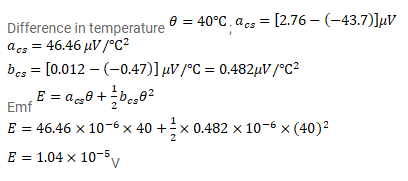# Find the thermo-emf developed in a copper-silver thermocouple

Question:

Find the thermo-emf developed in a copper-silver thermocouple when the junctions are kept at $0^{\circ} \mathrm{C}$ and $40^{\circ} \mathrm{C} .$ Use the data in table (33.1).

Solution: